Our World Statistics Day conversations have been a great reminder of how much statistics can inform our lives. Do you have an example of how statistics has made a difference in your life? Share your story with the Community!
Choose Language Hide Translation Bar
Highlighted

## Generalized Regression with calculated factors

I want to use the General Regression platform to compare different models.I have several factors, some of which a categorical and others a continuous numeric. I have normalized all factors to the interval [-1;1] and they are all calculated from other columns in the data table.

When I look at the models in the General Regression Platform, I get as expected models based on the calculated factors that I had selected in the model specification window of the Fit Model Platform: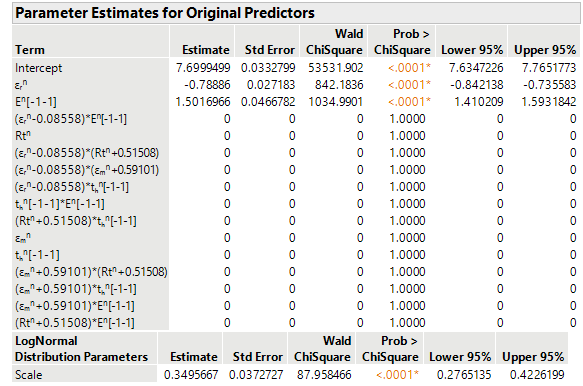Question: The Model Comparison table at the top of the Generalized Regression Platform indicates for this model "4 nonzero parameters" but I would only expect 3. How are the "nonzero parameters counted"?

When I put these models in the Formula Depot (Save Columns -> Publish Prediction Formula) the factors that are listed in the window are the columns that were used to calculate the parameters I use as input for the model fitting (like E^n), not the inputs themselves. (These are listed as "Others".)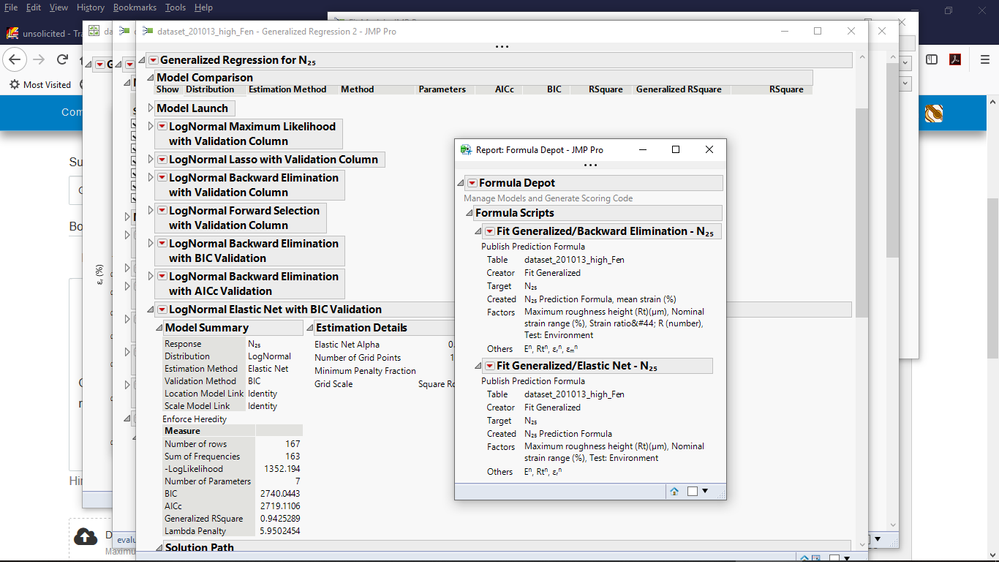Question: Is that the intended behaviour and is there a means to switch that off?

If I continue and use start the Model Comparison from the Formula Depot, I obtain the following window. On the top there is a question: "Which column is mean strain (%)_1 predicting?". In the original data table, there is a column mean strain(%) which is used to calculate one of the factors I want to put in the model. (There is no column mean strain (%)_1).

Question: What do I need to do in this window?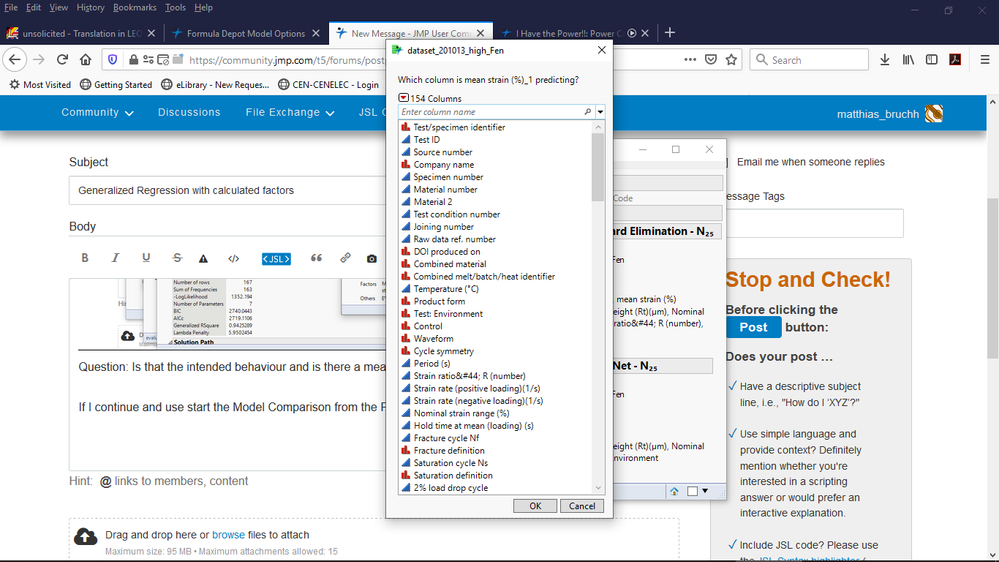For the time being I've selected the column εₘⁿ because that's the factor in the model that is calculated from the column mean strain(%).

Choosing the profile in the Model Comparison part of the Formula Depot I get this plot. "Mean strain" appears as output instead of input; what I want to predict is N25. So something seems to be completely wrong.

Question: What am I doing wrong?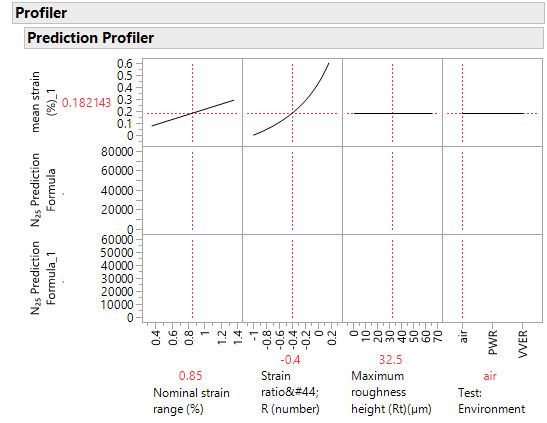The problem seem to disappear when I copy the calculated factors into a new table and start the model selection from there. So that's a workaround, but I loose the connection to the original table, so that's not an ideal solution.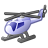peng_liu